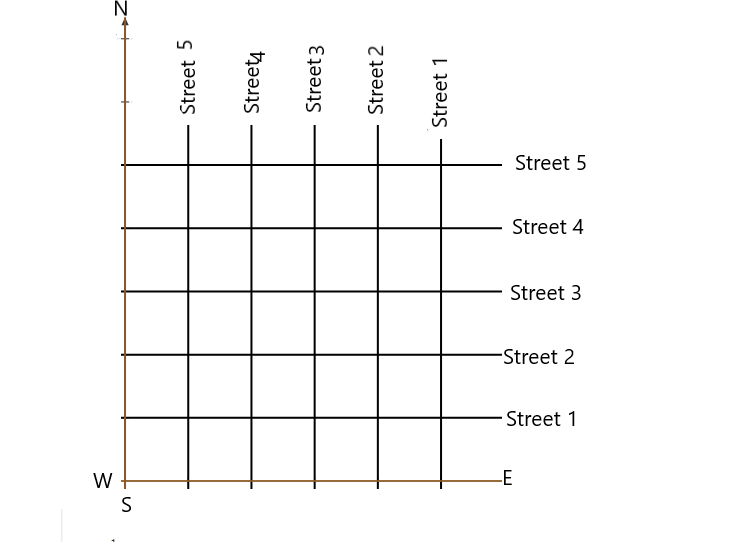# (Street Plan) : A city has two main roads which cross each other at the centre of the city. These two roads are along the North-South direction and East-West direction.All the other streets of the city run parallel to these roads and are $200 \mathrm{~m}$ apart. There are 5 streets in each direction. Using $1 \mathrm{~cm}=200 \mathrm{~m}$, draw a model of the city on your notebook. Represent the roads/streets by single lines.There are many cross- streets in your model. A particular cross-street is made by two streets, one running in the North - South direction and another in the East - West direction. Each cross street is referred to in the following manner : If the $2^{\text {nd }}$ street running in the North - South direction and $5^{\text {th }}$ in the East - West direction meet at some crossing, then we will call this cross-street $(2,5)$. Using this convention, find:(i) how many cross - streets can be referred to as $(4,3)$.(ii) how many cross - streets can be referred to as $(3,4)$.

To do:

We have to find

(i) The number of cross-streets that can be referred to as $(4,3)$.
(ii) The number of cross-streets that can be referred to as $(3,4)$.

Solution:

Draw two perpendicular lines as the two main roads of the city that cross each other at the centre.

Mark them as North-South (represented with N and S) and East-West (represented with E and W).

Given scale is $1\ cm = 200\ m$.

Draw five streets parallel to both the main roads.

Therefore,

The street plan is shown in the below figure.From the above graph, we can conclude that

(i) Only one street can be referred to as $(4, 3)$

(ii) Only one street can be referred to as $(3, 4)$.